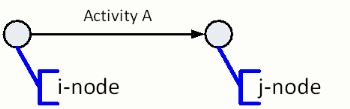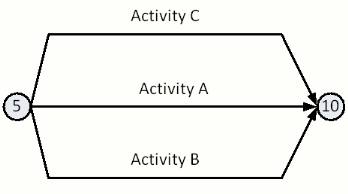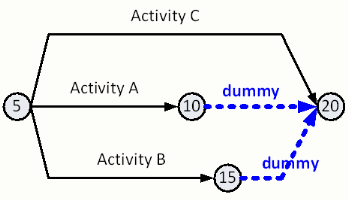# numbering dummies

While using names of activities is a good way for people to talk about a network, this is not the case for computer software used to work with networks. The first software program developed for arrow diagrams was created on mainframe computers using procedural programming languages. As a result of the software and hardware configurations that limited the original arrow diagram programmers.

The computer notation used for Arrow Diagrams was derived from the operations research formulation of acyclic networks. A frequently used notation in mathematics is the summation sign. When using the summation sign we often talk about summing from "i" to "j." This summation notation is the basis for numbering activities on arrow diagrams.

Each activity on an arrow diagram is identified according to a pair of numbers that list the node at the tail of an activity's arrow and the node at the head of an activity arrow. The node at the tail, or start of the activity, is called the "i-node." The node at the head, or end of the activity, is called the "j-node." The figure below shows the i- and j-nodes for single activity. If another activity is added at the end of an activity, then the j-node of the activity would be the i-node for the following activity.These nodes are labeled with node numbers. A rule when creating the node numbers is that the i-node must always be less than the j-node. Creating a schedule in this way will keep Arrow Diagrams from having a loop. The figure below shows example node numbers for Activity A.In large networks, activities are referred to using the shorthand notation provided by their i-node and j-node numbers. In order not to mistake one activity for another a unique pair of i-node and j-node numbers is required for every activity.

Since activities in networks share i-nodes and j-nodes, the unique designation of each activity may not be possible using the standard Arrow Diagram that we know so far. The figure below shows an example of the difficulties that encountered in numbering i-nodes and j-nodes.Numbering dummies are used when activities have the same node number pair. The Figure below numbering dummies are used for Activity A and Activity B to insure that all three activities in the network have unique node number pairs.Numbering Activity Diagrams occasionally causes problems for those learning this method. There are two key points to remember when numbing activities.

• Every activity must have a pair of unique numbers. If more than one activity with the same numbered node pair, then a numbering dummy must be added.
• There must be no loops in the Arrow Diagram. Always number the j-node larger than the i-node and you can avoid this problem.